```What products are involved?
This article is based on the use of RCP (Rheonics Control Panel) connected to the SME (Smart Module Electronics) from SRV, SRD, DVP and DVM. Hence it can be used for any of these Rheonics sensors.
```
`What is the purpose of this article?Explain the process to get a temperature compensated viscosity when it has a second degree polynomial trend. Usual applications are: Thermal oils and molten salts.`

#### To compensate for temperature effects, we need a mathematical model that can be based on Exponential, Polynomial or Arrhenius functions.

`2. Math model`

#### Along with the reference temperature, the reference viscosity (viscosity at Tref) is also of relevance for the calculations explained below.

`3. Checking the data`

#### 3.1. Select the reference temperature and viscosity from data obtained in tests. Here, reference temperature and viscosity are highlighted in blue in the next Table, we want to create a correlation at 20°C where we expect a viscosity of 1mPa.s.

 Temperature (°C) Viscosity (mPa.s) 30 0.75 25 0.85 20 1 15 1.1 10 1.25 5 1.5 0 1.75
Table 1. Data points from inline measurements.

3.2. Plot data points of Viscosity vs Temperature to study the behavior of the data. In this scenario, the data behavior can be represented by a second order polynomial curve.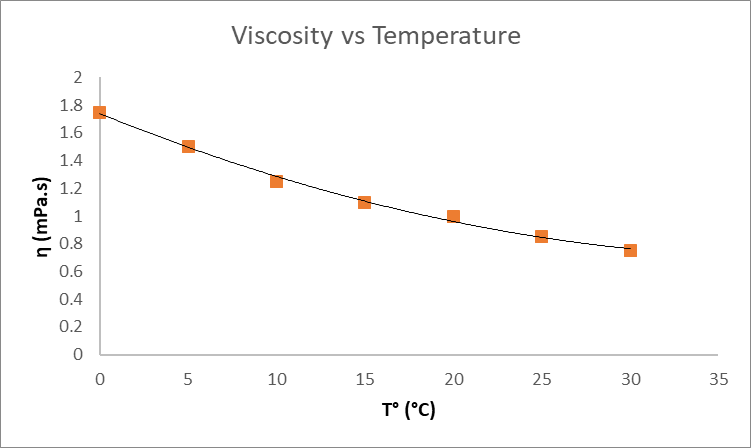3.3. Calculate the relation between compensated or reference viscosity and the measured viscosity by dividing the two.

 V' = (Compensated Viscosity / Viscosity) @20°C Results V' = (1/0.75) 1.3333 V' = (1/0.85) 1.1764 V' = (1/1) 1.0000 V' = (1/1.1) 0.9091 V' = (1/1.25) 0.8000 V' = (1/1.5) 0.6667 V' = (1/1.75) 0.5714

Table 2. Compensated viscosity calculation table.

3.4. Calculate the difference between measured and reference temperature by subtracting them.

 T' = T - Tref Results T' = 30-20 10 T' = 25-20 5 T' = 20-20 0 T' = 15-20 -5 T' = 10-20 -10 T' = 5-20 -15 T' = 0-20 -20

Table 3. Variation to the reference temperature.

3.5. Plot the V’ (Comp. Visco/Visco @20°) vs T' (T-Tref) and use a second order polynomial trendline to find X1 and X2 values or make a regression analysis to find the coefficients.

 V' T' 1.3333 10 1.1764 5 1.0000 0 0.9091 -5 0.8000 -10 0.6667 -15 0.5714 -20

Table 4. Datapoints for viscosity and temperature referenced to known values

#### A graph tool is used to create a trendline with the points obtained, it can also show the trendline equation.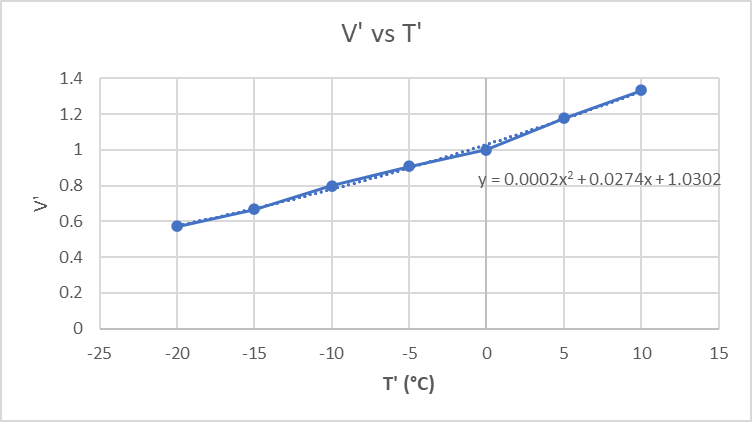Figure 2: Plot chart V' vs T'.

#### With all the input parameters set on the SME through the RCP software, the temperature compensated viscosity will look like next graph in Figure 3.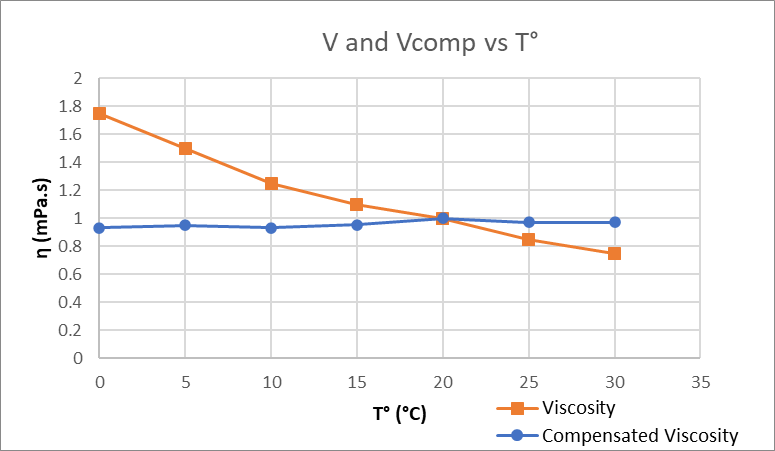Figure 3. Expected results when applying the mathematical model.

`4. How to load models into the SME?`

#### The calculation tab on RCP allows configuring the SME to run mathematical models for viscosity, density, and concentration. Expert mode needs to be enabled for this.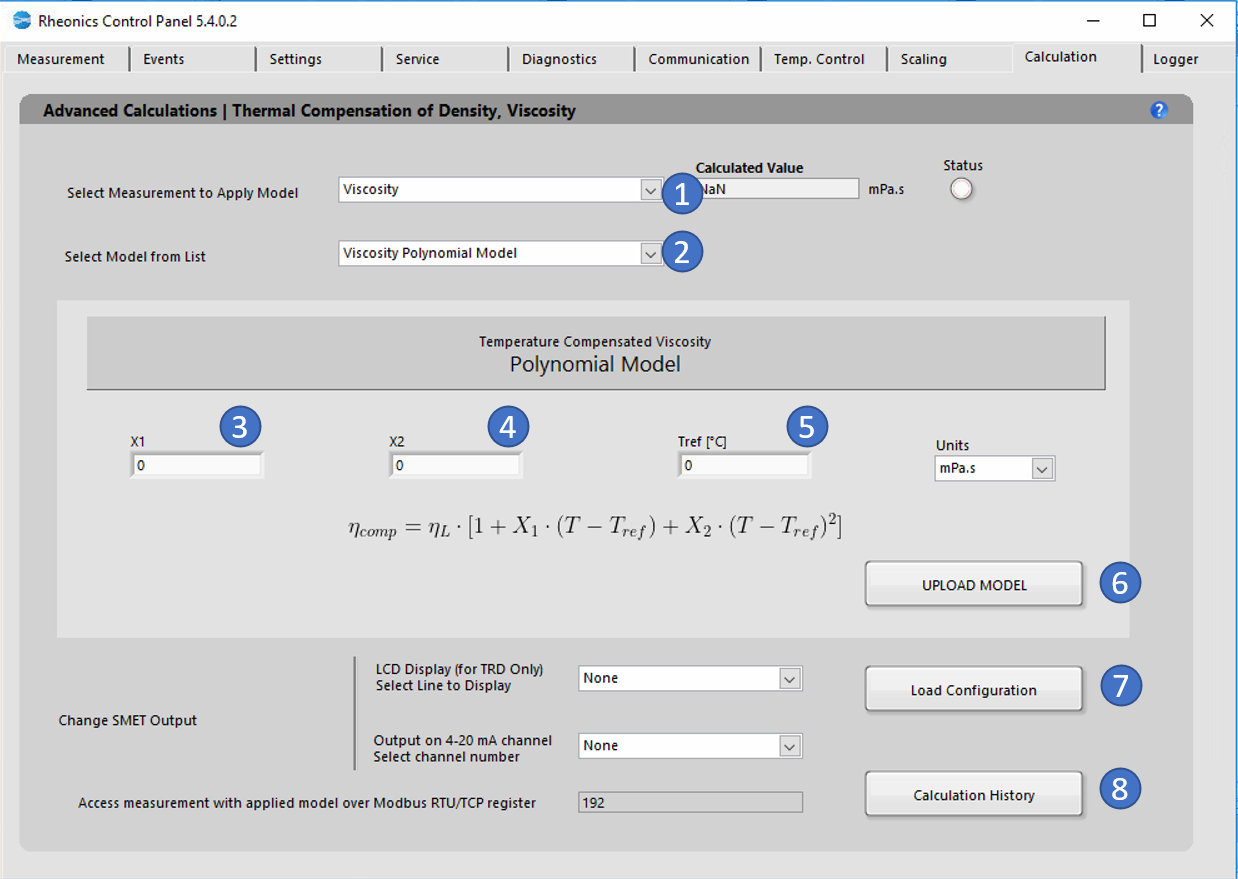Figure 4. Calculation tab for the exponential model of viscosity.

#### For the SME-TRD, the compensated viscosity values can be shown on the display. Select the line to display the parameter: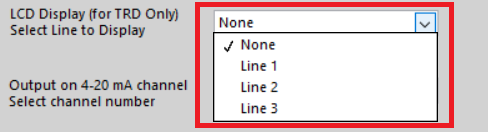Figure 5. Selecting read line for LCD display.

#### Changes can be verified on the “Communication” Tab.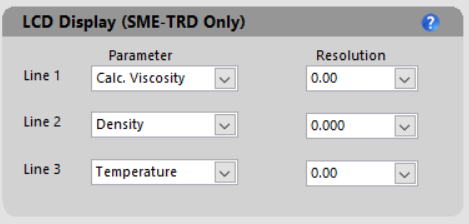Figure 6. The communication tab has loaded parameter.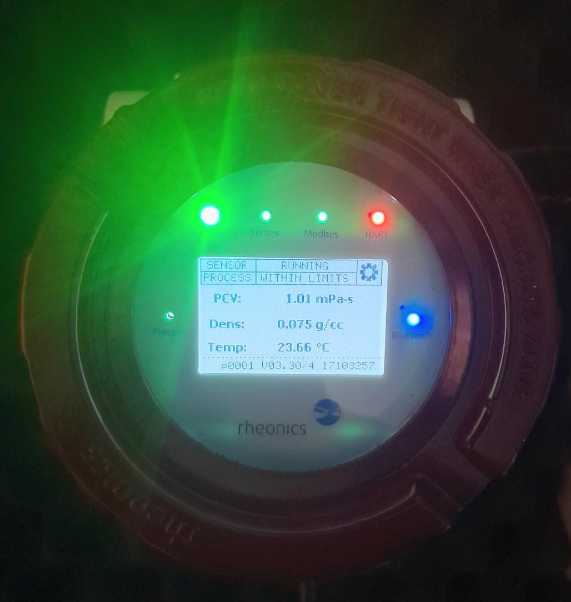Figure 7. Parameters loaded into the SMET-TRD.